第十七节，受限玻尔兹曼机网络及代码实现

"受限波尔兹曼"这名字听起来就霸气，算法如其名，也挺难的。之所以难，是因为我们大部分人都没学过概率图模型，其实RBM是条件随机场的变体，所以如果学习这个算法，建议先把CRF给熟悉了，那么学起来就会轻松很多。受限玻尔兹曼机是由Geoff Hinton发明，是一种用于降维、分类、回归、协同过滤、特征学习和主题搭建的算法。RBM网络作为一种无监督学习的方法，其目的是尽可能地表达输入数据的的规则和特征。

一 网络结构

RBM是有两个层的浅层神经网络，它是组成深度置信网络的基础部件。RBM的第一个层称为可见层，又称输入层，由显元 (visible units) 组成，用于输入训练数据。第二个层是隐藏层，相应地，由隐元 (hidden units) 组成，用作特征检测器 (feature detectors)。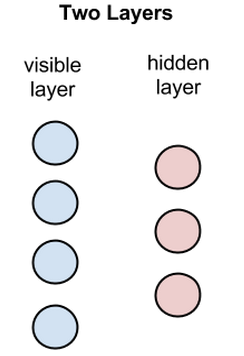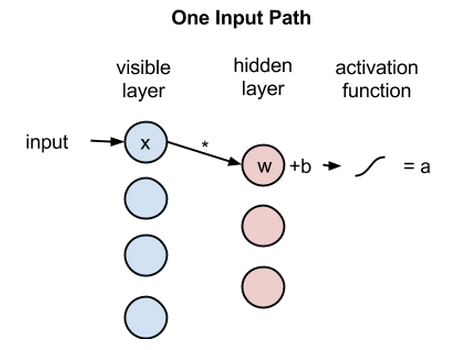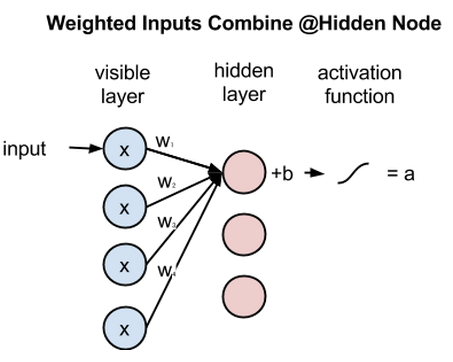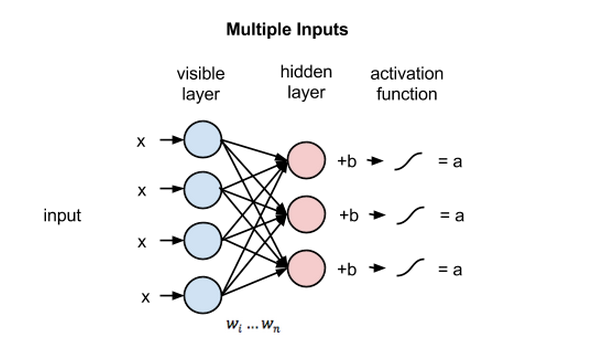二 重构• RBM在正向传递中使用输入值来预测节点的激活值，即输入为x时输出a的概率：p(a|x:w)。
• 但在反向传播时，激活值成为输入，而输出的是对于原始数据的重构值，或者说猜测值，此时RBM则是在尝试估计激活值为a时输入为x的概率，激活值得加权系数与正向传播中的权重相同。第二个阶段可以表示为：p(x|a:w)。

KL散度衡量两条曲线下方不重叠(即散度)的面积，而RBM的优化算法会尝试将这些离散部分的面积最小化，使共用权重在与第一层的激活值相乘后，可以得到与原始输入高度近似的结果。下图左半边是一组原始输入的概率分布曲线ρ，与之并列的是重构值的概率分布曲线q，右半边的图则显示了两条曲线之间的差异。RBM根据权重产生的误差反复调整权重，以此学习估计原始数据的近似值。可以说权重会慢慢开始反映出输入的结构，而这种结构被编码为第一个隐藏层的激活值。整个学习过程看上去像是两条概率分布曲线在逐步重合。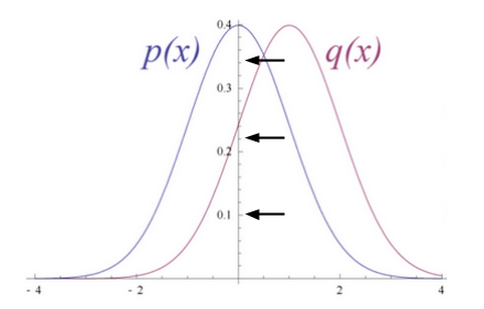三 使用 RBM 的过程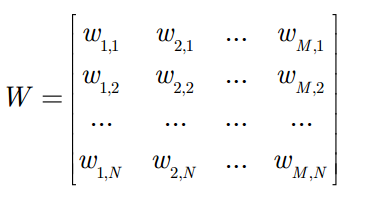• 首先，将每个隐藏节点的激励值 (activation) 计算出来：注意，这里用到了前面提到的神经元之间的条件独立性。
• 然后，将每个隐藏节点的激励值都用 S 形函数进行标准化，变成它们处于开启状 (用 1 表示) 的概率值：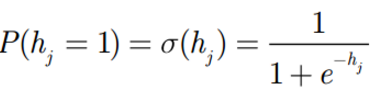• 至此，每个隐藏节点hj开启的概率被计算出来了。其处于关闭状态 (用 0 表示) 的概率自然也就是• 通常RBM中的神经元都是二值化的，也就是说只有开启和不开启两种状态，也就是0或者1。那么到底这个元开启还是关闭，我们需要将开启的概率与一个从 0, 1 均匀分布中抽取的随机值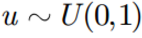进行如下比较四 训练 RBM

RBM 的训练过程，实际上是求出一个最能产生训练样本的概率分布。也就是说，要求一个分布，在这个分布里，训练样本的概率最大。由于这个分布的决定性因素在于权值W ，所以我们训练 RBM 的目标就是寻找最佳的权值。为了保持读者的兴趣，这里我们不给出最大化对数似然函数的推导过程，直接说明如何训练 RBM。

G. Hinton 提出了名为对比散度 (Contrastive Divergence) 的学习算法。下面我们来详述它的具体过程。 我们沿用前文的符号记法。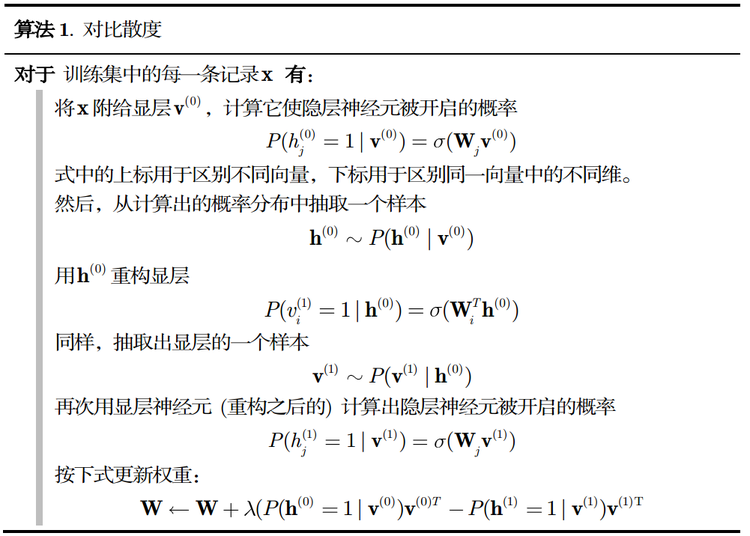五 实例代码

我们以MNIST数据集为例，进行训练，并打印出部分原始图像，以及重构后的图像，进行对比。程序中为了计算方便，我们的权重形状选择的是NxM，也就是之前假设的W的转置。
# -*- coding: utf-8 -*-
"""
Created on Sat May 19 09:30:02 2018

@author: zy
"""

'''

'''
import matplotlib.pylab as plt
import numpy as np
import random

class RBM(object):
'''
定义一个RBM网络类
'''

def __init__(self,n_visible,n_hidden,momentum=0.5,learning_rate=0.1,max_epoch=50,batch_size=128,penalty=0,weight=None,v_bias=None,h_bias=None):
'''
RBM网络初始化

使用动量的随机梯度下降法训练网络
args:
n_visible:可见层节点个数
n_hidden：隐藏层节点个数
momentum:动量参数 一般取值0.5,0.9,0.99  当取值0.9时，对应着最大速度1/(1-0.9)倍于梯度下降算法
learning_rate：学习率
max_epoch：最大训练轮数
batch_size：小批量大小
penalty：规范化 权重衰减系数  一般设置为1e-4  默认不使用
weight：权重初始化参数，默认是n_hidden x n_visible
v_bias:可见层偏置初始化 默认是 [n_visible]
h_bias:隐藏层偏置初始化 默认是 [n_hidden]
'''
#私有变量初始化
self.n_visible = n_visible
self.n_hidden = n_hidden
self.max_epoch = max_epoch
self.batch_size = batch_size
self.penalty = penalty
self.learning_rate = learning_rate
self.momentum = momentum

if weight is None:
self.weight = np.random.random((self.n_hidden,self.n_visible))*0.1        #用于生成一个0到0.1的随机符点数
else:
self.weight = weight
if v_bias is None:
self.v_bias = np.zeros(self.n_visible)                                    #可见层偏置
else:
self.v_bias = v_bias
if h_bias is None:
self.h_bias = np.zeros(self.n_hidden)                                     #隐藏层偏置
else:
self.h_bias = h_bias

def sigmoid(self,z):
'''
定义s型函数

args:
z：传入元素or list 、nparray
'''
return 1.0/(1.0+np.exp(-z))

def forword(self,inpt):
'''
正向传播

args:
inpt : 输入数据(可见层) 大小为batch_size x n_visible
'''
z = np.dot(inpt,self.weight.T) + self.h_bias        #计算加权和
return self.sigmoid(z)

def backward(self,inpt):
'''
反向重构

args:
inpt : 输入数据(隐藏层) 大小为batch_size x n_hidden
'''
z = np.dot(inpt,self.weight) + self.v_bias    #计算加权个
return self.sigmoid(z)

def batch(self):
'''
把数据集打乱，按照batch_size分组
'''
#获取样本个数和特征个数
m,n = self.input_x.shape

#生成打乱的随机数
per = list(range(m))
random.shuffle(per)

per = [per[k:k+self.batch_size] for k in range(0,m,self.batch_size)]

batch_data = []
for group in per:
batch_data.append(self.input_x[group])
return batch_data

def fit(self,input_x):
'''
开始训练网络

args:
input_x:输入数据集
'''
self.input_x = input_x

Winc = np.zeros_like(self.weight)
binc = np.zeros_like(self.v_bias)
cinc = np.zeros_like(self.h_bias)

#开始每一轮训练
for epoch in range(self.max_epoch):

batch_data = self.batch()
num_batchs = len(batch_data)

#存放平均误差
err_sum = 0.0

#随着迭代次数增加 penalty减小
self.penalty = (1 - 0.9*epoch/self.max_epoch)*self.penalty

#训练每一批次数据集
for v0 in  batch_data:
'''
RBM网络计算过程
'''
#前向传播  计算h0
h0 = self.forword(v0)
h0_states = np.zeros_like(h0)
#从 0, 1 均匀分布中抽取的随机值，尽然进行比较判断是开启一个隐藏节点，还是关闭一个隐藏节点
h0_states[h0 > np.random.random(h0.shape)] = 1
#print('h0',h0.shape)

#反向重构  计算v1
v1 = self.backward(h0_states)
v1_states = np.zeros_like(v1)
v1_states[v1 > np.random.random(v1.shape)] = 1
#print('v1',v1.shape)

#前向传播 计算h1
h1 = self.forword(v1_states)
h1_states = np.zeros_like(h1)
h1_states[h1 > np.random.random(h1.shape)] = 1
#print('h1',h1.shape)

'''更新参数 权重和偏置  使用栋梁的随机梯度下降法'''
#计算batch_size个样本的梯度估计值
dW = np.dot(h0_states.T , v0) - np.dot(h1_states.T , v1)
#沿着axis=0进行合并
db = np.sum(v0 - v1,axis=0).T
dc = np.sum(h0 - h1,axis=0).T

#计算速度更新
Winc = self.momentum  * Winc + self.learning_rate * (dW  - self.penalty * self.weight)/self.batch_size
binc = self.momentum  * binc + self.learning_rate * db / self.batch_size
cinc = self.momentum  * cinc + self.learning_rate * dc / self.batch_size

#对于最大化对数似然函数  使用梯度下降法是加号 最小化是减号  开始更新
self.weight = self.weight + Winc
self.v_bias = self.v_bias + binc
self.h_bias = self.h_bias + cinc

err_sum = err_sum + np.mean(np.sum((v0 - v1)**2,axis=1))

#计算平均误差
err_sum = err_sum /num_batchs
print('Epoch {0},err_sum {1}'.format(epoch, err_sum))

def predict(self,input_x):
'''
预测重构值

args:
input_x：输入数据
'''
#前向传播  计算h0
h0 = self.forword(input_x)
h0_states = np.zeros_like(h0)
#从 0, 1 均匀分布中抽取的随机值，尽然进行比较判断是开启一个隐藏节点，还是关闭一个隐藏节点
h0_states[h0 > np.random.random(h0.shape)] = 1

#反向重构  计算v1
v1 = self.backward(h0_states)
return v1

def visualize(self, input_x):
'''
传入 形状为m xn的数据 即m表示图片的个数  n表示图像的像素个数

其中 m = row x row
n = s x s

args:
input_x:形状为 m x n的数据
'''
#获取输入样本的个数和特征数
m, n = input_x.shape

#获取每张图像的宽和高 默认宽=高
s = int(np.sqrt(n))

#把所有图片以 row x row排列
row = int(np.ceil(np.sqrt(m)))

#其中多出来的row + 1是用于绘制边框的
data = np.zeros((row*s + row + 1, row * s + row + 1)) - 1.0

#图像在x轴索引
x = 0
#图像在y轴索引
y = 0
#遍历每一张图像
for i in range(m):
z = input_x[i]
z = np.reshape(z,(s,s))
#填充第i张图像数据
data[x*s + x + 1 :(x+1)*s + x + 1 , y*s + y + 1 :(y+1)*s + y + 1] = z
x = x + 1
#换行
if(x >= row):
x = 0
y = y + 1
return data

'''
加载数据集  数据按行分割，每一行表示一个样本，每个特征使用空格分割

args:
path：数据文件路径
'''
data = []
for line in open(path, 'r'):
ele = line.split(' ')
tmp = []
for e in ele:
if e != '':
tmp.append(float(e.strip(' ')))
data.append(tmp)
return data

if __name__ == '__main__':
#加载MNIST数据集 总共有5000张图像，每张图像有784个像素点   MNIST数据集可以从网上下载
data = np.array(data)
print(data.shape)                  #(5000, 784)

#创建RBM网络
rbm = RBM(784, 100,max_epoch = 50,learning_rate=0.05)
#开始训练
rbm.fit(data)

#显示64张手写数字
images = data[0:64]
print(images.shape)
a = rbm.visualize(images)
fig = plt.figure(1,figsize=(8,8))
plt.imshow(a,cmap=plt.cm.gray)
plt.title('original data')

#显示重构的图像
rebuild_value = rbm.predict(images)
b = rbm.visualize(rebuild_value)
fig = plt.figure(2,figsize=(8,8))
plt.imshow(b,cmap=plt.cm.gray)
plt.title('rebuild data')

#显示权重
w_value = rbm.weight
c = rbm.visualize(w_value)
fig = plt.figure(3,figsize=(8,8))
plt.imshow(c,cmap=plt.cm.gray)
plt.title('weight value(w)')
plt.show()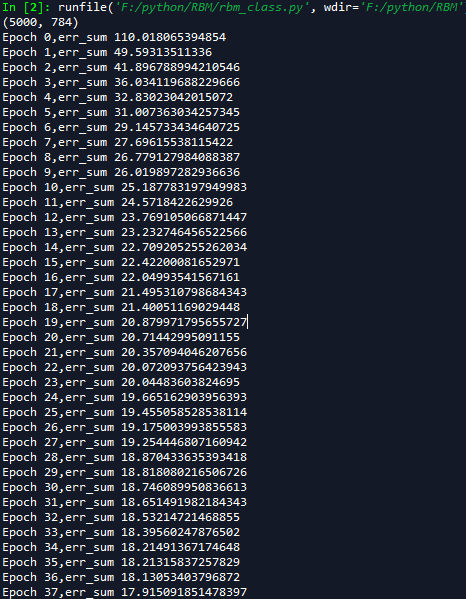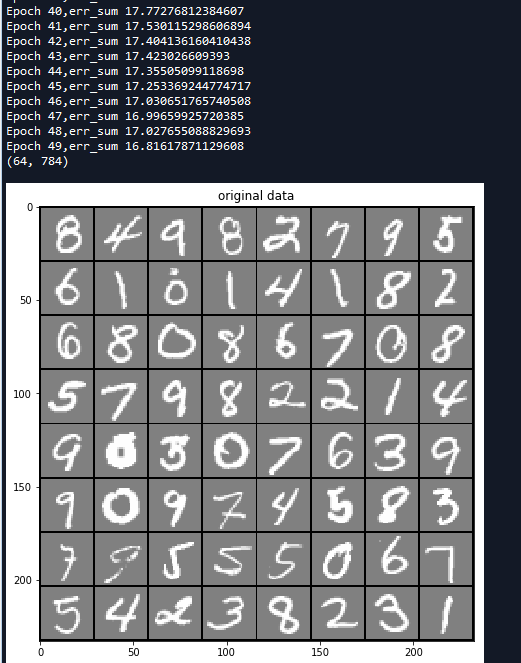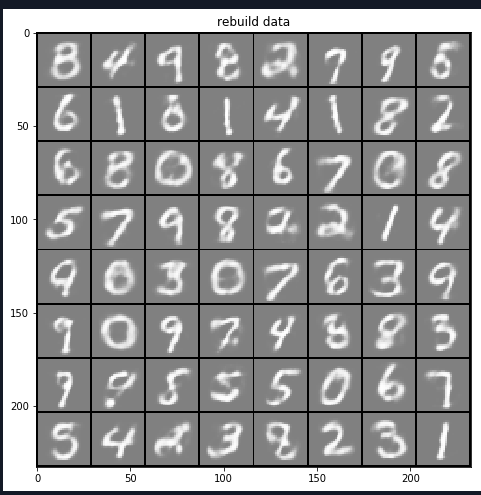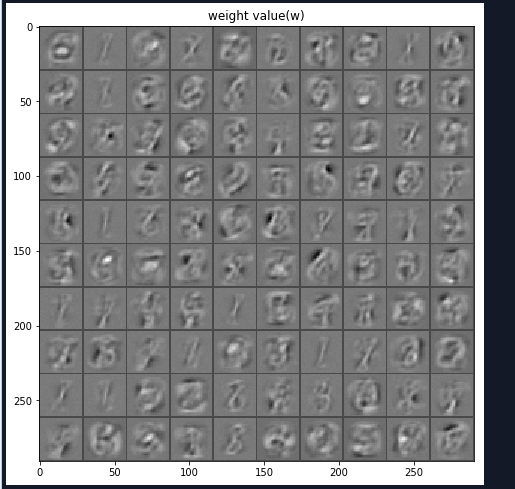Andrew Ng机器学习笔记（三）（拓展）深度学习与受限玻尔兹曼机(推荐)

受限玻尔兹曼机基础教程

深度信念网络与受限玻尔兹曼机

受限玻尔兹曼机——简单证明

简单易学的机器学习算法——受限玻尔兹曼机RBM

受限的玻尔兹曼机

受限玻尔兹曼机（Restricted Boltzmann Machine，RBM）

深度学习（八）RBM受限波尔兹曼机学习-未完待续

posted @ 2018-05-19 17:10  大奥特曼打小怪兽  阅读(4332)  评论(3编辑  收藏  举报